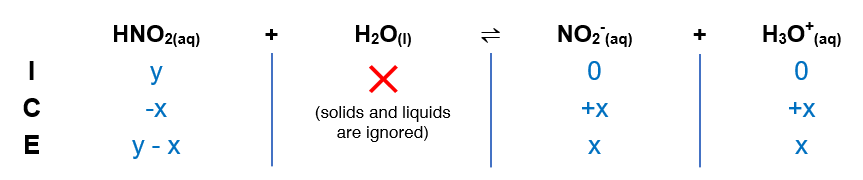# Problem: Determine the pH of an HNO2 solution of each of the following concentrations. In which cases can you not make the simplifying assumption that x is small?a. 0.500 Mb. 0.100 Mc. 0.0100 M

###### FREE Expert Solution

For the pH of each HNO2 solution:

Ka of HNO2 → 4.0x10-4

(1) Construct an ICE table (where y is the concentration of HNO2)(2) Calculate the [H3O+] using Ka expression

Inspecting each concentration of HNO2:###### Problem Details

Determine the pH of an HNO2 solution of each of the following concentrations. In which cases can you not make the simplifying assumption that x is small?
a. 0.500 M
b. 0.100 M
c. 0.0100 M